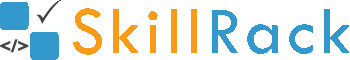Must Solve 100 Programs for Campus Placement Part - 7/104 months ago

1) Characters - Position Times

The program must accept a string S of length 5 as the input. The program must print the first character once, the second character two times, the third character three times, the fourth character four times and the fifth character five times as the output.

Example Input/Output 1:
Input:
match

Output:
maatttcccchhhhh

Click the link below to solve this program
http://www.skillrack.com/solve/8106

2) Two Integer Triangle Pattern

The program must accept two integers N1 and N2 as the input. The program must print the desired pattern as shown in the Example Input/Output section.

Example Input/Output 1:
Input:
5 8

Output:
5 6 7 8
5 6 7
5 6
5

Example Input/Output 2:
Input:
1 5

Output:
1 2 3 4 5
1 2 3 4
1 2 3
1 2
1

Click the link below to solve this program
http://www.skillrack.com/solve/8110

3) First Three Factors

A non prime number N is passed as the input to the program. The program must print the first three factors of N as the output.

Example Input/Output 1:
Input:
9

Output:
1 3 9

Click the link below to solve this program
http://www.skillrack.com/solve/8103

4) Sort - Same Unit Digit

The program must accept three integers X, Y and Z as the input. Two of these three integers will have same unit digit. The program must print the smallest integer among the integers which are having the same unit digit. Then the program must print the largest integer among the integers which are having the same unit digit. Finally, the program must print the remaining integer as the output.

Example Input/Output 1:
Input:
72 29 12

Output:
12 72 29

Example Input/Output 2:
Input:
10 5 90

Output:
10 90 5

Click the link below to solve this program
http://www.skillrack.com/solve/8109

5) 12 to 24 Hour Time Format

The program must accept the time in 24-hour format. The program must print the time in 12-hour format as the output.

Example Input/Output 1:
Input:
0000

Output:
12:00 AM

Click the link below to solve this program
http://www.skillrack.com/solve/8108

6) Element in Xth Position

The program must accept N integers and an integer X as the input. The program must print the Xth integer as the output.

Boundary Condition(s):
1 <= N <= 100
1 <= X <= N

Example Input/Output 1:
Input:
5
10 52 63 74 90
3

Output:
63

Click the link below to solve this program
http://www.skillrack.com/solve/9230

7) Reverse N Integers

The program must accept N integers as the input. The program must print the N integers in reverse order as the output.

Example Input/Output 1:
Input:
6
10 22 30 14 51 60

Output:
60 51 14 30 22 10

Example Input/Output 2:
Input:
8
12 86 94 36 58 62 44 72

Output:
72 44 62 58 36 94 86 12

Click the link below to solve this program
http://www.skillrack.com/solve/9228

8) Print Two Digit Numbers

The program must accept N integers as the input. The program must print all the two digit integers among the N integers in reverse order as the output. If there is no two digit integer then the program must print -1 as the output.

Boundary Condition(s):
1 <= N <= 100
1 <= Each integer value <= 10^6

Example Input/Output 1:
Input:
6
10 987 75 1011 5 99

Output:
99 75 10

Example Input/Output 2:
8
203 540 897 1 6 501 150 2

Output:
-1

Click the link below to solve this program
http://www.skillrack.com/solve/9229

9) Product - Last Digit & First Digit

The program must accept two integers X and Y as the input. The program must print the product of the last digit of X and the first digit of Y as the output.

Example Input/Output 1:
Input:
514 2004

Output:
8

Example Input/Output 2:
Input:
56 375

Output:
18

Click the link below to solve this program
http://www.skillrack.com/solve/9227

10) 2 Power X

The program must accept an integer X as the input. The program must print the value of 2X (2 power X) as the output.

Example Input/Output 1:
Input:
4

Output:
16

Example Input/Output 2:
Input:
5

Output:
32

Click the link below to solve this program
http://www.skillrack.com/solve/9226